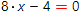Solve linear equation online

Linear equation is the equation of the form:

a∙x + b = c

The linear equation always has one root only. The equation entered into calculator in its natural form. Decimal numbers, fractions and even parameters can be used as coefficients. In the latter case, solution will be given in the common form.

Linear equations solverLinear equation you want to solve: